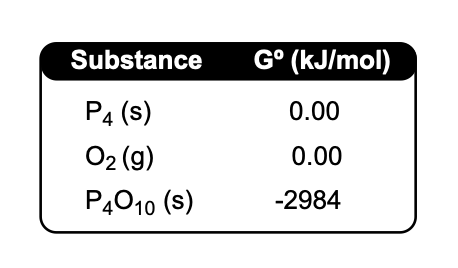Clutch Prep is now a part of Pearson
Ch.18 - ElectrochemistryWorksheetSee all chapters

# Cell Potential: G and K

See all sections
Sections
Standard Reduction Potentials
Intro to Electrochemical Cells
Galvanic Cell
Electrolytic Cell
Cell Potential: Standard
Cell Potential: The Nernst Equation
Cell Potential and Gibbs Free Energy
Cell Potential and Equilibrium
Cell Potential: G and K
Cell Notation
Electroplating

Cell Potential, Gibbs Free Energy and the Equilibrium Constant deal with the spontaneity of electrochemical cells

###### The Triangle Diagram

Concept #1: The relationship between these 3 variables and spontaneity can be observed through the Triangle Diagram.

Example #1: Calculate the standard cell potential for the following reaction if 10 moles of electrons are transferred.

P4 (s) + 5 O2 (g)  →  P4O10 (s)Practice: Given the following standard reduction potentials, determine Ksp for Hg2Cl2(s) at 25 °C.

Hg22+ (aq) + 2 e  →. 2 Hg (l)                                                     E°red = + 0.789 V

Hg2Cl(s) + 2 e  →. 2 Hg (l) + 2 Cl – (aq)                                 E°red = + 0.271 V

Practice: What is the value of the cell potential for the 4 electron transfer reaction below if the equilibrium mixture contains 0.255 M of CH4, 1.10 M CO2, 0.388 M CO and 0.250 M Hat 25ºC?

CH4 (g) + CO2 (g)  ⇌. 2 CO (g) + 2 H2 (g)

Practice: Given the reaction: 2 Cl2 (g) + 2 H2O (g)  ⇌  4 HCl (g) + O2 (g) Kp = 7.5x10-2, calculate the Gibbs Free Energy change for the reaction below at 30ºC.

8 HCl (g) + 2 O2 (g)  ⇌ 4 Cl2 (g) + 4 H2O (g)